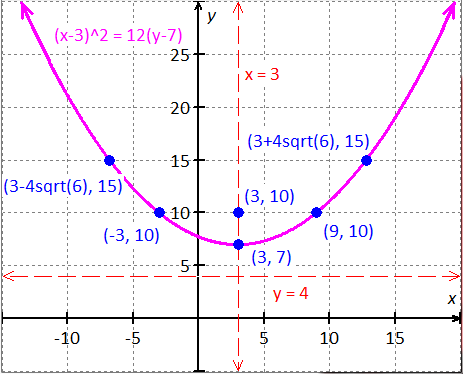PRE-ALGEBRA
ALGEBRA 1
GEOMETRY
ALGEBRA 2
TRIGONOMETRY
PRE-CALCULUS
CALCULUSPrecalculus 2014PAGE: 428 SET: Exercises PROBLEM: 1
Please look in your text book for this problem StatementThe equation is.

Since the square term is, the parabola is vertical.

Standard form of the vertical parabola is,

whereis vertex.

Focus.

Axis of symmetry.

Directrix.

The parabola equation.Compare the above equation with.

Since, the parabola opens up.

VertexFocusAxis of symmetryDirectrix.

Graph the vertex, focus, axis of symmetry and directrix.

Construct a table of values to graph the general shape of the curve..

Solve for..Plot the points and connect the curve.

Graph:

Graph of:Vertex.

Focus.

Axis of symmetry.

Directrix.

Graph of:.TESTIMONIALS
 "I want to tell you that our students did well on the math exam and showed a marked improvement that, in my estimation, reflected the professional development the faculty received from you. THANK YOU!!!" June Barnett "Your site is amazing! It helped me get through Algebra." Charles "My daughter uses it to supplement her Algebra 1 school work. She finds it very helpful." Dan Pease
 Tweets by @mathskeydotcom

QUESTIONS? LET US HELP.
mathskey.com is not affiliated with any Publisher, Book cover, Title, Author names appear for reference only